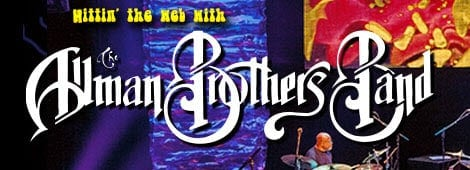Follow the instructions below for the genuine thrill that comes with discovering your blues name:

From the first list, take the name using the initial of your first name. From the second list, do the same with your middle name. From the third, your surname.

First List

A=Fat; B=Muddy ; C=Crippled; D=Old; E=Texas; F=Hollerin’; G=Ugly; H=Brown; I=Happy; J=Boney; K=Curly; L=Pretty; M=Jailhouse; N=Peg Leg; O=Red; P=Sleepy; Q=Bald; R=Skinny; S=Blind; T=Big; U=Yella; V=Toothless; W=Screamin’; X=Fat Boy; Y=Washboard; Z=Steel-Eye

Second List

A=Bones; B=Money; C=Harp; D=Legs; E=Eyes; F=Lemon; G=Killer; H=Hips; I=Lips; J=Fingers; K=Boy; L=Liver; M=Gumbo; N=Foot; O=Mama; P=Back; Q=Duke; R=Dog; S=Bad Boy; T=Baby; U=Chicken; V=Pickles; W=Sugar; X=Cracker; Y=Tooth; Z=Smoke

Third List

A=Jackson; B=McGee; C=Hopkins; D=Dupree; E=Green; F=Brown; G=Jones; H=Rivers; I=Malone; J=Washington; K=Smith; L=Parker; M=Lee; N=Thompkins; O=King; P=Bradley; Q=Hawkins; R=Jefferson; S=Davis; T=Franklin; U=White; V=Jenkins; W=Bailey; X=Johnson; Y=Blue; Z=Allison